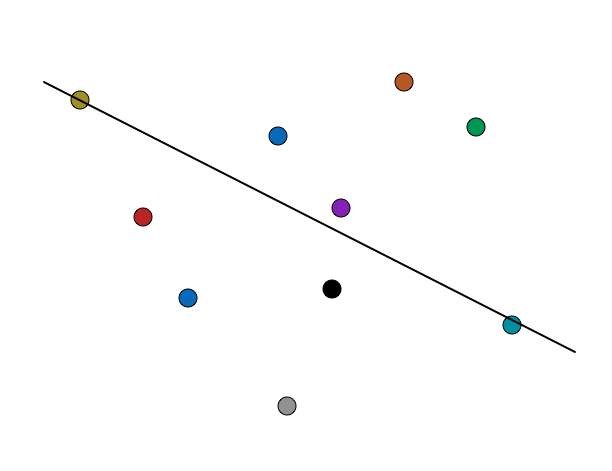# Equally Divided 10 Points

Probability Level 3Consider all set of ten points which lie in a plane and no three of them are collinear.

There are at least $x$ lines which pass through exactly two of these points, and divide the plane into 2 regions which each contain four of the remaining points.

What is the largest possible value of $x$?

×